• ------------------------- qq_plot(y) 显示 y 的样本分位数与正态分布的理论分位数分位数-分位数图。 如果 y 的分布是正态分布，则该图将接近线性。 qq_plot(x,y) 显示两个样本的分位数-分位数图。 如果样本来自...matlab
• ## Python计算分位数

万次阅读 多人点赞 2018-07-04 14:12:42
Python中可以利用Numpy库来计算分位数，示例如下：import numpy as np a = np.array([1, 2, 3, 4, 5, 6, 7, 8, 9, 10]) # 中位数 print(np.median(a)) # 25%分位数 print(np.percentile(a, 25)) # 75%分位数 print...
Python中可以利用Numpy库来计算分位数，示例如下：
import numpy as np

a = np.array([1, 2, 3, 4, 5, 6, 7, 8, 9, 10])
# 中位数
print(np.median(a))
# 25%分位数
print(np.percentile(a, 25))
# 75%分位数
print(np.percentile(a, 75))
输出结果：
5.5
3.25
7.75

展开全文Python
• 实现分布滞后分位数回归，包括方差标准差，t检验各系数是否显著
• ## 分位数计算

千次阅读 2019-09-22 18:42:44
分位数（Quantile），亦称分位点，是指将一个随机变量的概率分布范围分为几个等份的数值点，常用的有中位数（即二分位数）、四分位数、百分位数等。 网上看到的例子： [1,2,3,4,5,6,6,6,6,7,8,9]这个list的90分位数...
分位数（Quantile），亦称分位点，是指将一个随机变量的概率分布范围分为几个等份的数值点，常用的有中位数（即二分位数）、四分位数、百分位数等。
网上看到的例子：
[1,2,3,4,5,6,6,6,6,7,8,9]这个list的90分位数是7.9
计算原理：
首先将你的输入数组进行从小到大排序，然后计算:
(n−1)∗p=i+j
其中n为数组元素的个数，将计算结果的整数部分用i表示，小数部分用j来表示。则最终的percentile值为：
res=(1−j)∗array[i]+j∗array[i+1]
因为题目给的数组是排好序的，所以我们计算（n-1）*p = (12-1)*0.9 = 9.9，则整数部分 i=9，小数部分j=0.9。查找数组得知，array[i] = array = 7;array[i+1] = array = 8。
因为，我们可以得到测试样例的结果为：
res=(1−j)∗array[i]+j∗array[i+1]=(1−0.9)∗7+0.9∗8=7.9
我自己封装一个方法：
/**
* 计算分位数
* @param $data 计算分位数的数组，数组格式 * @param$number   第n个分位数;例如90分位数是9，10分位数是1
* @param $quantile_type 分位数形式; 例如四分位数是4，十分位数是10，百分位数是100 * @return float|int|mixed|string */ public function getQuantile($data, $number,$quantile_type)
{
sort($data);$count = count($data); // 数组内个数$quantile_interval = ($count - 1) /$quantile_type;  // 计算分位间
$nth_number = 1 +$number * $quantile_interval; // 计算第n个数的数字$nth_floor = floor($nth_number); // 第n个数的数字的前一位数$nth_ceil = ceil($nth_number) + 1; // 第n个数的数字的后一位数$quantile_proportion = preg_replace('/'.$nth_floor.'/', '0',$nth_number, 1);   // 第n个数字的小数
$nth_floor_number =$data[$nth_floor-1]; // 第n个数的数字的前一位数对应数组中的值$nth_ceil_number = $data[$nth_ceil-1];  // 第n个数的数字的后一位数对应数组中的值
$quantile =$nth_floor_number + ($nth_ceil_number -$nth_floor_number) * $quantile_proportion; // 计算分位数 return$quantile;
}

直接使用即可，分位数在多领域都被应用，有兴趣的小伙伴建议多研究研究的！
展开全文php 排序
• ## 分位数

千次阅读 2020-05-22 10:49:44
分位数（Quantile），亦称分位点，是指将一个随机变量的概率分布范围分为几个等份的数值点，常用的有中位数（即二分位数）、四分位数、百分位数等。 常见分类 1.二分位数 对于有限的数集，可以通过把所有观察值高低...
分位数（Quantile），亦称分位点，是指将一个随机变量的概率分布范围分为几个等份的数值点，常用的有中位数（即二分位数）、四分位数、百分位数等。
常见分类 1.二分位数 对于有限的数集，可以通过把所有观察值高低排序后找出正中间的一个作为中位数。如果观察值有偶数个，则中位数不唯一，通常取最中间的两个数值的平均数作为中位数，即二分位数。 一个数集中最多有一半的数值小于中位数，也最多有一半的数值大于中位数。如果大于和小于中位数的数值个数均少于一半，那么数集中必有若干值等同于中位数。 计算有限个数的数据的二分位数的方法是：把所有的同类数据按照大小的顺序排列。如果数据的个数是奇数，则中间那个数据就是这群数据的中位数；如果数据的个数是偶数，则中间那2个数据的算术平均值就是这群数据的中位数。
2.四分位数 四分位数（Quartile）是统计学中分位数的一种，即把所有数值由小到大排列并分成四等份，处于三个分割点位置的数值就是四分位数。 1）第一四分位数(Q1)，又称“较小四分位数”，等于该样本中所有数值由小到大排列后第25%的数字； 2）第二四分位数(Q2)，又称“中位数”，等于该样本中所有数值由小到大排列后第50%的数字； 3）第三四分位数(Q3)，又称“较大四分位数”，等于该样本中所有数值由小到大排列后第75%的数字。 第三四分位数与第一四分位数的差距又称四分位距。
3.百分位数 百分位数，统计学术语，如果将一组数据从小到大排序，并计算相应的累计百分位，则某一百分位所对应数据的值就称为这一百分位的百分位数。运用在教育统计学中，例如表现测验成绩时，称PR值。
四分位数的位置确定 Q1的位置= (n+1) × 0.25 Q2的位置= (n+1) × 0.5 Q3的位置= (n+1) × 0.75 n表示项数
分位数计算的算法：
将数组array从小到大排序，计算(n-1)*p的整数部分为i，小数部分为j，其中n为数组大小，则percentile的值是：(1-j)*array第i+1个数+j*array第i+2个数。
实例1
数据总量: 6, 47, 49, 15, 42, 41, 7, 39, 43, 40, 36 由小到大排列的结果: 6, 7, 15, 36, 39, 40, 41, 42, 43, 47, 49 一共11项 Q1 的位置=（11+1） × 0.25=3， Q2 的位置=（11+1）× 0.5=6， Q3的位置=（11+1） × 0.75=9
Q1 = 15， Q2 = 40， Q3 = 43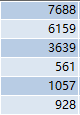数据升序排序的结果：
561，928，1057，3639，6159，7688
计算第二四分位数(Q2)
n = 6
(n-1)*p = (6-1)*0.5 = 2.5
整数部分i = 2， 小数部分为j=0.5
Q2 = (1-0.5)* 1057 + 0.5 * 3639
= 2348


展开全文• 分位数 定义：对随机变量X和给定的α\alphaα ，（0&amp;amp;lt;α\alphaα&amp;amp;lt;1）,若存在
分位数
定义：设随机变量X的分布函数为F(x),对任意给定的实数 p ，（0<p<1）,若存在

x

p

x_p

使得 P{

X

≤

X\leq

x

p

x_p

} = F(

x

p

x_p

) = p成立，那么称

x

p

x_p

为此概率分布的p分位数。 例：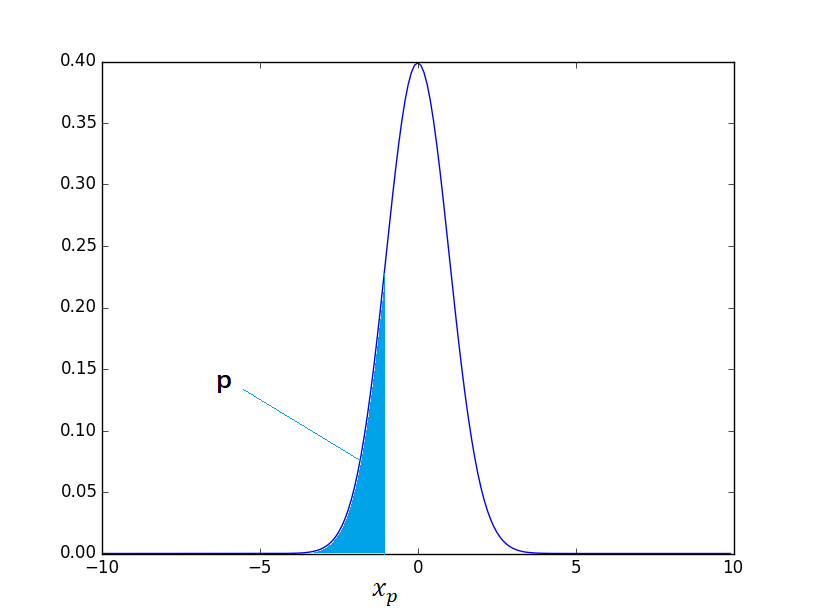如上图所示，图像为标准正态分布的概率密度函数，

x

p

x_p

为p分位数。
上侧分位数
定义：对随机变量X和给定的

α

\alpha

(0<

α

\alpha

<1),若存在

x

α

x_\alpha

,使得 P{X

≥

\geq

x

α

x_\alpha

} =

α

\alpha

,那么称

x

α

x_\alpha

为X的上侧分位数。 例：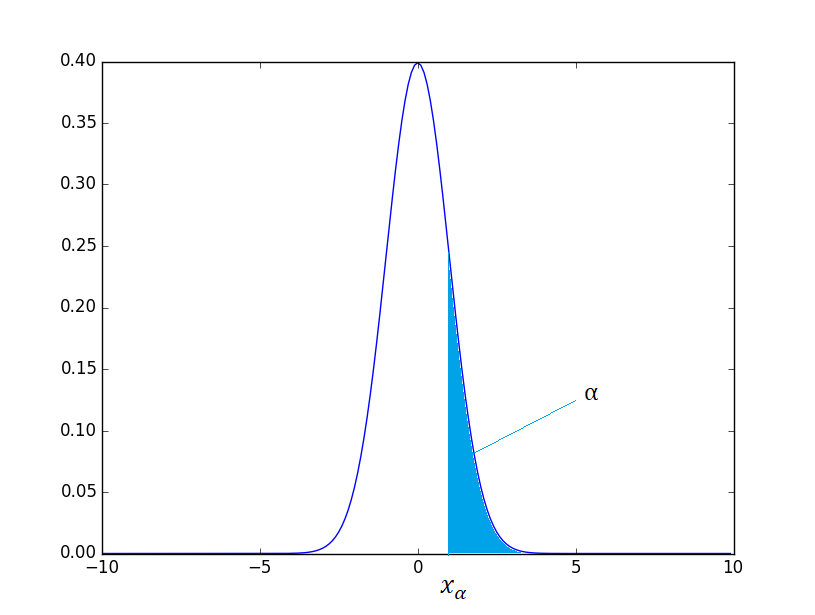如上图所示，

x

α

x_\alpha

为标准正态分布的上侧

α

\alpha

分位数。 在数理统计教程中，又把标准正态分布的上侧分位数记为：

u

α

u_\alpha

Python实现
首先介绍一下几个常用分布的函数 import scipy.stats as st st.norm() #正态分布 st.t() #t分布 st.f() #f分布 st.chi2() #

χ

2

\chi^2

分布 然后介绍一下基本的操作： pdf 概率密度函数 cdf 分布函数 ppf 分布函数的逆 sf 残损函数（1-cdf） isf 逆残损函数
下面是代码实现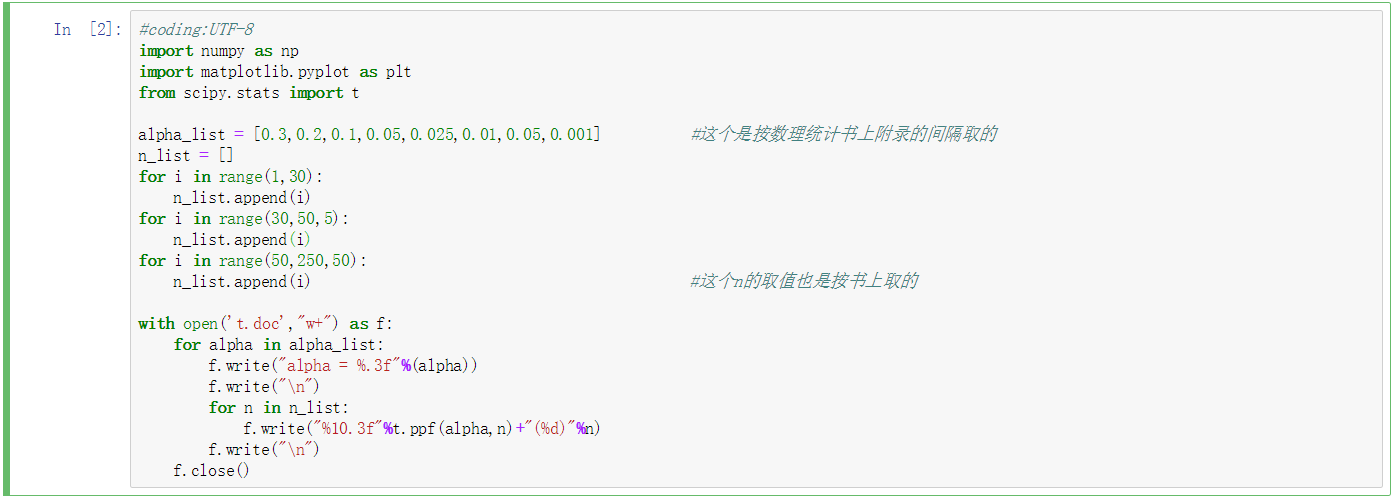看到结果，你可能会问，怎么跟数理统计书附录上的t分位数表不一样，是因为书上求的是上侧分位数表。
最后再画个图吧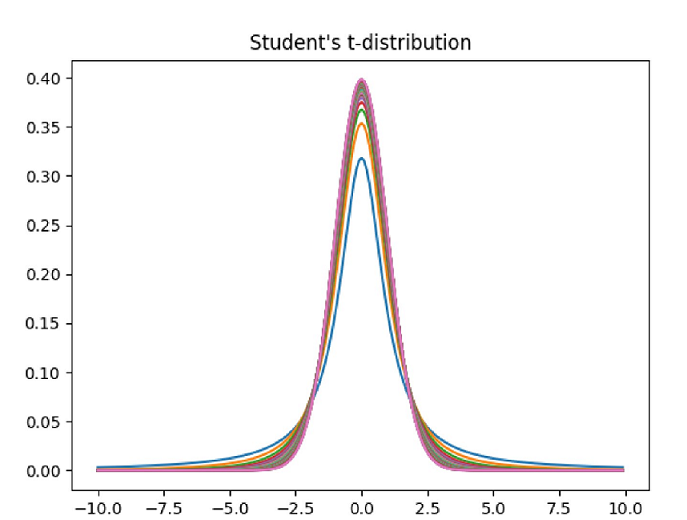展开全文• ## p分位数的原理及计算

万次阅读 多人点赞 2018-07-17 16:54:08
1.统计分位数概念 2.分位数的计算方法及举例 2.1首先确定p分位数的位置（依据项数分为基数、偶数情况） 2.2 求一步确定的p分位数位置处的具体值 3.python中的分位数计算 1.统计分位数概念 统计，...
• ## 分位数的简单理解

万次阅读 多人点赞 2017-05-05 15:25:19
在统计学里经常提到分位数的概念，下面我们通过一个例子来简单解释一下。假设有一千名学生参加了某次考试， 学生A得了75分，排名603，603/1000＝60.3% 学生B得了94分，排名28，28/1000=2.8% 此时，A大约在60.3%的...四分位
• 分位数回归matlab代码，绝对可用，有注释，以及运行时间显示
• 我的理解：分位数是以概率将一批数据进行分割 一、你首先得有数据 比如说，这里有一组6个同学的分数为[12, 31, 22, 61, 52, 88],然后我们将其由小到大排序[12, 22, 31, 52, 61, 88] 二、给定概率点求p分位数 ...
• # 求分位数 def get_quartiles(result_list,quartile_numer): ''' result_list：是待计算的分位数的数列，用list表示 quartile_numer：是要计算的分位数的比例 ''' result_list=sorted(result_list) num=len...
• 我们在进行分析过程中，经常会有计算某个特征的分位数这个需求。下面为大家介绍如何在oracle计算某一列数据的分位数。 需要求分位数的表结构如下： select * from test_lizhen;   我们发现该表有两列，一列...oracle
• CAViaR：基于分位数回归和条件自回归风险价值模型计算风险价值
• 分位数回归,分位数回归模型,Python源码.zip
• ## 分位数回归（Quantile Regression）

万次阅读 多人点赞 2019-07-09 22:25:16
在介绍分位数回归之前，先重新说一下回归分析，我们之前介绍了线性回归、多项式回归、核回归等等，基本，都是假定一个函数，然后让函数尽可能拟合训练数据，确定函数的未知参数。尽可能拟合训练数据，一般是通过...
• 1的p，称F=p的X为此分布的分位数，或者分位数。简单的说，分位数指的就是连续分布函数中的一个点，这个点的一侧对应概率p。 维基百科的解释：分位数（英语：Quantile），亦称分位点，是指用分割点（c
• ## R语言分位数

千次阅读 2020-10-13 00:17:57
0.05的上分位数与0.95的下分位数相同。 正态分布0.05的下分位数： qnorm(0.05,lower.tail=FALSE) 正态分布0.95的下分位数： qnorm(0.95,lower.tail=TRUE)
• 详细介绍了分位数回归的原理，并阐述了如何用Eviews实现
• ## 分位数回归-Quantile regression

万次阅读 多人点赞 2018-12-16 12:09:21
分位数回归是估计一组回归变量X被解释变量Y的分位数之间线性关系的建模方法。 以往的回归模型实际是研究被解释变量的条件期望。而人们也关心解释变量被解释变量分布的中位数，分位数呈何种关系。它最早由......